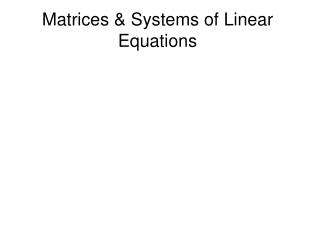DownloadDownload PresentationMatrices & Systems of Linear Equations

# Matrices & Systems of Linear Equations

Download Presentation## Matrices & Systems of Linear Equations

- - - - - - - - - - - - - - - - - - - - - - - - - - - E N D - - - - - - - - - - - - - - - - - - - - - - - - - - -
##### Presentation Transcript

1. Matrices & Systems of Linear Equations

2. Special Matrices

3. Special Matrices

4. Equality of Matrices Two matrices are said to be equal if they have the same size and their corresponding entries are equal

5. Equality of Matrices Use the given equality to find x, y and z

6. Matrix Addition and SubtractionExample (1)

7. Matrix Addition and SubtractionExample (2)

8. Multiplication of a Matrix by a Scalar

9. Matrix Multiplication(n by m) Matrix X (m by k) MatrixThe number of columns of the matrix on the left= number of rows of the matrix on the right The result is a (n by k) Matrix

10. Matrix Multiplication3x3 X 3x3

11. Matrix Multiplication1x3 X 3x3→ 1x3

12. Example (1)

13. Example (2)(1X3) X (3X3) → 1X3

14. Example (3)(3X1) X (1X2) → 3X2

15. Example (4)

16. Transpose of Matrix

17. Properties of the Transpose

18. Matrix ReductionDefinitions (1) 1. Zero Row:A row consisting entirely of zeros 2. Nonzero Row:A row having at least one nonzero entry 3. Leading Entry of a row:The first nonzero entry of a row.

19. Matrix ReductionDefinitions (2) Reduced Matrix: A matrix satisfying the following: 1. All zero rows, if any, are at the bottom of the matrix 2. The leading entry of a row is 1 3. All other entries in the column in which the leading entry is located are zeros. 4. A leading entry in a row is to the right of a leading entry in any row above it.

20. Examples of Reduced Matrices

21. Examples matrices that are not reduced

22. Elementary Row Operations 1. Interchanging two rows 2. Replacing a row by a nonzero multiple of itself 3. Replacing a row by the sum of that row and a nonzero multiple of another row.

23. Interchanging Rows

24. Replacing a row by a nonzero multiple of itself

25. Replacing a row by the sum of that row and a nonzero multiple of another row

26. Augmented Matrix Representing a System of linear Equations

27. Solving a System of Linear Equations by Reducing its Augmented Matrix Using Row Operations

28. Solution

29. Solution of the System

30. The Idea behind the Reduction Method

31. Interchanging the First & the Second Row

32. Multiplying the first Equation by 1/3

33. Adding to the Third Equation 12 times the Second Equation

34. Dividing the Third Equation by 40

35. Adding to the First Equation 7 times the third Equation

36. Systems with infinitely many Solutions

37. Systems with infinitely many Solutions